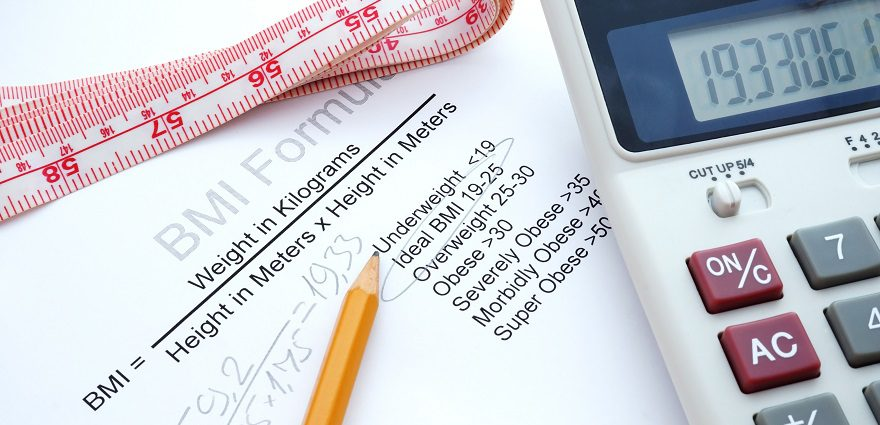s

# Measuring Obesity with the Body Mass IndexHow do we measure obesity? Body mass index (BMI) is a good indicator, because it correlates pretty well with the amount of body fat a person has. BMI is a formula that calculates the ratio between height and weight:

• Measuring using pounds and inches:
(pounds of weight / [inches of height]2) x 703
For example, if your weight is 170 lbs. and your height is 5’6″ (66″), then the calculation is: (170 ÷ 662) x 703 = 27.4
• Measuring using kilograms and meters:
kilograms of weight / meters of height2
For example, if your weight is 75 kg and your height is 162 cm (1.62 m), then the calculation is: 75 ÷ 1.622 = 28.6

If you don’t want to do the math, use the handy calculator below.

The body mass index range for adults 20 and over is shown here:

BMI Weight Status
Below 18.5 Underweight
18.5 – 24.9 Normal
25.0 – 29.9 Overweight
30.0 and above Obese

Your physician can use your BMI to help determine if you have a weight problem. But a high BMI isn’t enough information to determine a course of action. For example, healthy athletes may weigh more than other people of the same height because they have well-developed muscles; muscle tissue is denser than fat tissue, so a particular volume of muscle will weigh more than the same volume of fat.

Your doctor will need to evaluate your diet, how much exercise you get, your family history of disease and many other factors before determining the next step for you.